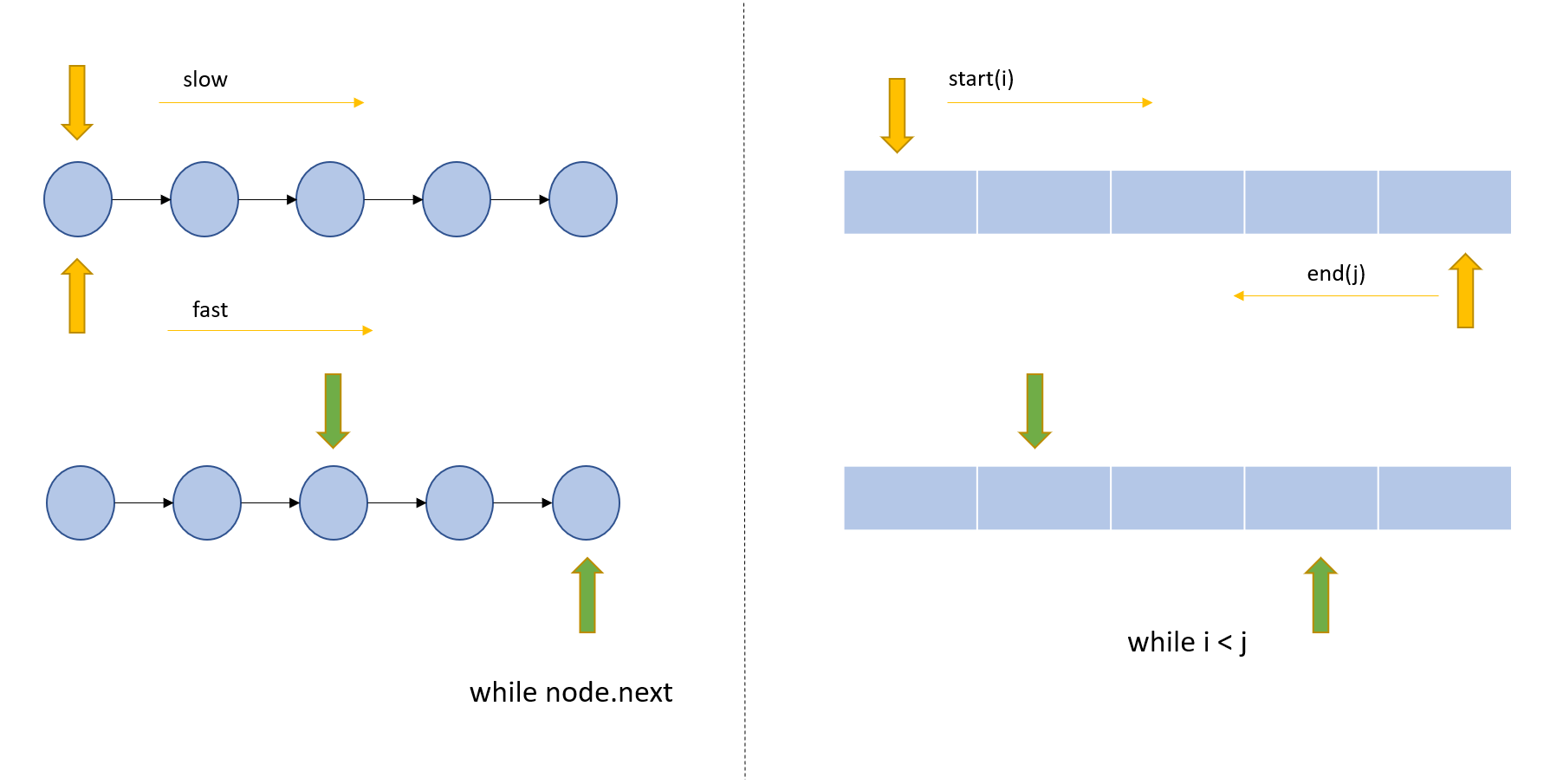# Two Pointers Approach — Python CodeIn this tutorial, we'll learn Two Pointers Approach — Python Code.

Two are better than one if they act as one.

Two pointer algorithm is one of the most commonly asked questions in any programming interview. This approach optimizes the runtime by utilizing some order (not necessarily sorting) of the data. It is generally applied on lists (arrays) and linked lists. This is generally used to search pairs in a sorted array. This approach works in constant space.

In this technique pointers represent either index or an iteration attribute like node’s next.

### Steps in two pointer approach:Image by author

As in the above pic, the two-pointer approach has three main steps:

Pointer Initialization — Starting points. Pointers can be at any place depending upon what we are trying to achieve. In the left part of the pic, we have both pointers starting at the same position i.e. start of the linked list. In the right part of the pic, we have pointers at extreme ends one at starting index and another one at the last index.

*Pointer movement — *This will decide how we converge towards the solution. Pointer can move in the same direction (left in above pic) or they can move in the opposite direction (right in the above pic). Also in the left part of the pic, we have different increments for the pointers(top (slow) with 1 unit bottom (fast) with 2 units).

*Stop condition — *This decides when do we stop. In the left part, we continue till we reach a node whose next element is None. In the right one, we continue till our start is less than the end (i <j).

Note: Sliding window is another variant of two pointer approach.

Let see how we use the above logic by solving some problems.

## top 30 Python Tips and Tricks for Beginners

In this post, we'll learn top 30 Python Tips and Tricks for Beginners

## 50+ Basic Python Code Examples

In this tutorial, we'll learn 50+ Basic Python Code Examples.List, strings, score calculation and more..

## Guide to Python Programming Language

Guide to Python Programming Language

## Lambda, Map, Filter functions in python

You can learn how to use Lambda,Map,Filter function in python with Advance code examples. Please read this article

## Using Black To Auto Format Your Python

In this post, we'll learn how to stop worrying and embrace Auto Formatting in your python code.